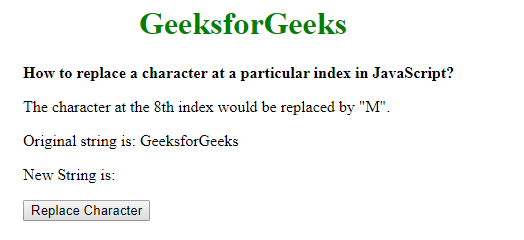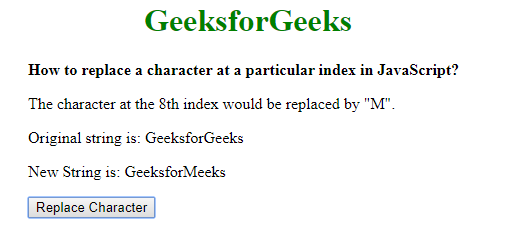# How to replace a character at a particular index in JavaScript ?

To replace a character from a string there are popular methods available, the two most popular methods we are going to describe in this article. The first method is by using the substr() method. And in the second method, we will convert the string to an array and replace the character at the index. Both methods are described below:

Using the substr() method: The substr() method is used to extract a sub-string from a given starting index to another index. This can be used to extract the parts of the string excluding the character to be replaced.

The first part of the string can be extracted by using the starting index parameter as ‘0’ (which denotes the starting of the string) and the length parameter as the index where the character has to be replaced.

The second part of the string can be extracted by using the starting index parameter as ‘index + 1’, which denotes the part of the string after the index of the character. The second parameter is omitted to get the whole string after it.

The new string created concatenating the two parts of the string with the character to be replaced added in between. This will create a new string with the character replaced at the index.

• Syntax:
```function replaceChar(origString, replaceChar, index) {
let firstPart = origString.substr(0, index);
let lastPart = origString.substr(index + 1);

let newString = firstPart + replaceChar + lastPart;
return newString;
}
```
• Example:

 ` ` `<``html``> ` ` `  `<``head``> ` `    ``<``title``> ` `        ``How to replace a character at a ` `        ``particular index in JavaScript? ` `    `` ` `     `  `    ``<``style``> ` `        ``.container{ ` `            ``text-align: left; ` `            ``width:500px; ` `            ``padding-left:60px; ` `        ``} ` ` `  `    `` ` ` ` ` `  `<``body``> ` `    ``<``center``> ` `        ``<``h1` `style``=``"color: green"``> ` `            ``GeeksforGeeks ` `        `` ` `         `  `        ``<``div` `class``=``"container"``> ` `            ``<``b``> ` `                ``How to replace a character at a ` `                ``particular index in JavaScript? ` `            `` ` `             `  `            ``<``p``> ` `                ``The character at the 8th index ` `                ``would be replaced by "M". ` `            `` ` `             `  `            ``<``p``> ` `                ``Original string is: GeeksforGeeks ` `            `` ` `             `  `            ``<``p``> ` `                ``New String is: ` `                ``<``span` `class``=``"output"``> ` `            `` ` `         `  `            ``<``button` `onclick``=``"changeText()"``> ` `                ``Replace Character ` `            `` ` `        `` ` `    `` ` `     `  `    ``<``script` `type``=``"text/javascript"``> ` `        ``function replaceChar(origString, replaceChar, index) ` `        ``{ ` `            ``let firstPart = origString.substr(0, index); ` `             `  `            ``let lastPart = origString.substr(index + 1); ` ` `  `            ``let newString =  ` `                ``firstPart + replaceChar + lastPart; ` `             `  `            ``return newString; ` `        ``} ` ` `  `        ``function changeText() { ` `            ``originalText = "GeeksforGeeks"; ` ` `  `            ``charReplaced = ` `                ``replaceChar(originalText, "M", 8); ` ` `  `            ``document.querySelector('.output').textContent ` `                    ``= charReplaced; ` `        ``} ` `    `` ` ` ` ` `  ` `

• Output:
Before Clicking the button:After Clicking the button:Converting the string to an array and replacing the character at the index: The string is converted to an array using the split() method with the separator as a blank character (“”). This will split the string into an array and make every character accessible as an index of the array.

The character which has to replaced can then be simply assigned to the corresponding index of the array. The array is joined back into a string using the join() method with the separator as a blank character (“”). This will create a new string with the character replaced at the index.

• Syntax:
```function replaceChar(origString, replaceChar, index) {
let newStringArray = origString.split("");

newStringArray[index] = replaceChar;

let newString = newStringArray.join("");

return newString;
}```
• Example:

 ` ` `<``html``> ` ` `  `<``head``> ` `    ``<``title``> ` `        ``How to replace a character at a ` `        ``particular index in JavaScript? ` `    `` ` `     `  `    ``<``style``> ` `        ``.container { ` `            ``text-align: left; ` `            ``width: 500px; ` `            ``padding-left: 60px; ` `        ``} ` `    `` ` ` ` ` `  `<``body``> ` `    ``<``center``> ` `        ``<``h1` `style``=``"color: green"``> ` `            ``GeeksforGeeks ` `        `` ` `         `  `        ``<``div` `class``=``"container"``> ` `            ``<``b``> ` `                ``How to replace a character at a ` `                ``particular index in JavaScript? ` `            `` ` `             `  `            ``<``p``> ` `                ``The character at the 8th index ` `                ``would be replaced by "M". ` `            `` ` `             `  `            ``<``p``> ` `                ``Original string is: GeeksforGeeks ` `            `` ` `             `  `            ``<``p``> ` `                ``New String is: ` `                ``<``span` `class``=``"output"``> ` `            `` ` ` `  `            ``<``button` `onclick``=``"changeText()"``> ` `                ``Replace Character ` `            `` ` `        `` ` `    `` ` `     `  `    ``<``script` `type``=``"text/javascript"``> ` `        ``function replaceChar(origString, replaceChar, index) ` `        ``{ ` `            ``let newStringArray = origString.split(""); ` ` `  `            ``newStringArray[index] = replaceChar; ` ` `  `            ``let newString = newStringArray.join(""); ` ` `  `            ``return newString; ` `        ``} ` ` `  `        ``function changeText() { ` `             `  `            ``originalText = "GeeksforGeeks"; ` ` `  `            ``charReplaced = ` `                ``replaceChar(originalText, "M", 8); ` ` `  `            ``document.querySelector('.output').textContent ` `                        ``= charReplaced; ` `        ``} ` `    `` ` ` ` ` `  ` `

• Output:
Before Clicking button:After Clicking button:My Personal Notes arrow_drop_upIm listening

If you like GeeksforGeeks and would like to contribute, you can also write an article using contribute.geeksforgeeks.org or mail your article to contribute@geeksforgeeks.org. See your article appearing on the GeeksforGeeks main page and help other Geeks.

Please Improve this article if you find anything incorrect by clicking on the "Improve Article" button below.